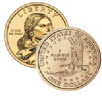# Probability

How likely something is to happen.

Many events can't be predicted with total certainty. The best we can say is how likely they are to happen, using the idea of probability.

### Tossing a CoinWhen a coin is tossed, there are two possible outcomes:

• heads (H) or
• tails (T)

We say that the probability of the coin landing H is ½

And the probability of the coin landing T is ½

### Throwing DiceWhen a single die is thrown, there are six possible outcomes: 1, 2, 3, 4, 5, 6.

The probability of any one of them is 16

## Probability

In general:

Probability of an event happening = Number of ways it can happen Total number of outcomes

### Example: the chances of rolling a "4" with a die

Number of ways it can happen: 1 (there is only 1 face with a "4" on it)

Total number of outcomes: 6 (there are 6 faces altogether)

So the probability = 1 6

### Example: there are 5 marbles in a bag: 4 are blue, and 1 is red. What is the probability that a blue marble gets picked?

Number of ways it can happen: 4 (there are 4 blues)

Total number of outcomes: 5 (there are 5 marbles in total)

So the probability = 4 5 = 0.8

## Probability Line

We can show probability on a Probability Line:Probability is always between 0 and 1

## Probability is Just a Guide

Probability does not tell us exactly what will happen, it is just a guide

### Example: toss a coin 100 times, how many Heads will come up?

Probability says that heads have a ½ chance, so we can expect 50 Heads.

But when we actually try it we might get 48 heads, or 55 heads ... or anything really, but in most cases it will be a number near 50.

## Words

Some words have special meaning in Probability:

Experiment: a repeatable procedure with a set of possible results.### Example: Throwing dice

We can throw the dice again and again, so it is repeatable.

The set of possible results from any single throw is {1, 2, 3, 4, 5, 6}

Outcome: A possible result of an experiment.### Example: Getting a "6"

Sample Space: all the possible outcomes of an experiment.### Example: choosing a card from a deck

There are 52 cards in a deck (not including Jokers)

So the Sample Space is all 52 possible cards: {Ace of Hearts, 2 of Hearts, etc... }

The Sample Space is made up of Sample Points:

Sample Point: just one of the possible outcomes

### Example: Deck of Cards

• the 5 of Clubs is a sample point
• the King of Hearts is a sample point

"King" is not a sample point. There are 4 Kings, so that is 4 different sample points.### Example: Throwing dice

There are 6 different sample points in the sample space.Event: one or more outcomes of an experiment

### Example Events:

An event can be just one outcome:

• Getting a Tail when tossing a coin
• Rolling a "5"

An event can include more than one outcome:

• Choosing a "King" from a deck of cards (any of the 4 Kings)
• Rolling an "even number" (2, 4 or 6)

Hey, let's use those words, so you get used to them:### Example: Alex wants to see how many times a "double" comes up when throwing 2 dice.

The Sample Space is all possible Outcomes (36 Sample Points):

{1,1} {1,2} {1,3} {1,4} ... {6,3} {6,4} {6,5} {6,6}

The Event Alex is looking for is a "double", where both dice have the same number. It is made up of these 6 Sample Points:

{1,1} {2,2} {3,3} {4,4} {5,5} and {6,6}

These are Alex's Results:

Experiment Is it a Double?
{3,4} No
{5,1} No
{2,2} Yes
{6,3} No
... ...

After 100 Experiments, Alex has 19 "double" Events ... is that close to what you would expect?